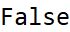# Wolfram CloudResource Object

Function Resource

# SophieGermainPrimeQ

Determines if the given number is a Sophie Germain prime, or not

 ResourceFunction["SophieGermainPrimeQ"][p] gives True if p is a Sophie Germain prime and False otherwise.

## Details and Options

A Sophie Germain prime is a prime number q, where 2q+1 is also prime.

## Examples

### Basic Examples

The prime number 11 is a Sophie Germain prime, because both 11 and 23 (2·11+1) are primes:

 In:=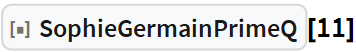Out=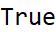The number 14 is not a Sophie Germain prime, because it is not a prime itself, even though 29 (2·14+1) is a prime number:

 In:=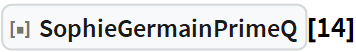Out=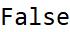The number 7 is not a Sophie Germain prime, because 15 (2·7+1) is not prime:

 In:=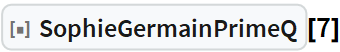Out=